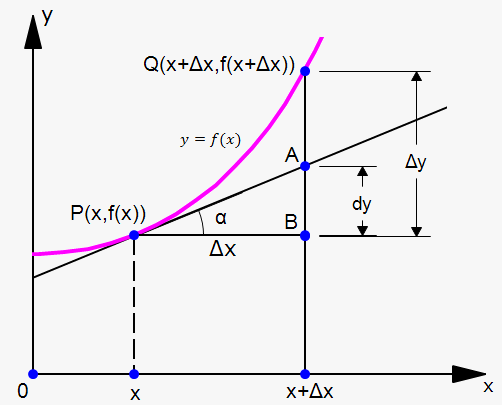# Differentials

## Verwandter Rechner: Rechner für lineare Näherung

Suppose that we are given a function $y={f{{\left({x}\right)}}}$. Consider the interval ${\left[{a},{a}+\Delta{x}\right]}$. The corresponding change in ${y}$ is $\Delta{y}={f{{\left({a}+\Delta{x}\right)}}}-{f{{\left({a}\right)}}}$.

We are interested in the following question: is there a constant $C$ such that $\Delta{y}\approx{C}\Delta{x}$ when $\Delta{x}\to{0}$?

Let's go through a couple of examples.

Example 1. The area of a circle with the radius $r$ is $A=\pi{{r}}^{{2}}$.

If we increase $r$ by $\Delta{r}$, then the corresponding increase in ${A}$ is $\Delta{A}=\pi{{\left({r}+\Delta{r}\right)}}^{{2}}-\pi{{r}}^{{2}}={2}\pi{r}\Delta{r}+{{\left(\Delta{r}\right)}}^{{2}}$.

When $\Delta{r}\to{0}$, we have that ${{\left(\Delta{r}\right)}}^{{2}}$ becomes very small, so we can write that $\Delta{A}\approx{2}\pi{r}\Delta{r}$.

Let's move on.

Example 2. The volume of a sphere with the radius ${r}$ is ${V}=\frac{{4}}{{3}}\pi{{r}}^{{3}}$.

If we increase ${r}$ by $\Delta{r}$, then the corresponding increase in ${V}$ is $\Delta{V}=\frac{{4}}{{3}}\pi{{\left({r}+\Delta{r}\right)}}^{{3}}-\frac{{4}}{{3}}\pi{{r}}^{{3}}={4}\pi{{r}}^{{2}}\Delta{r}+{4}\pi{r}{{\left(\Delta{r}\right)}}^{{2}}+\frac{{4}}{{3}}\pi{{\left(\Delta{r}\right)}}^{{3}}$.

When $\Delta{r}\to{0}$, we have that both ${{\left(\Delta{r}\right)}}^{{2}}$ and ${{\left(\Delta{r}\right)}}^{{3}}$ become very small, so we can write that $\Delta{V}\approx{4}\pi{{r}}^{{2}}\Delta{r}$.

In the above examples, we can see the same thing.

In Example 1, when ${A}=\pi{{r}}^{{2}}$, we have that $\Delta{A}\approx{2}\pi{r}\Delta{r}$, but ${2}\pi{r}$ is the derivative of $\pi{{r}}^{{2}}$ with respect to ${r}$.

In Example 2, when ${V}=\frac{{4}}{{3}}\pi{{r}}^{{3}}$, we have that $\Delta{V}\approx{4}\pi{{r}}^{{2}}\Delta{r}$, but again, ${4}\pi{{r}}^{{2}}$ is the derivative of $\frac{{4}}{{3}}\pi{{r}}^{{3}}$ with respect to ${r}$.

In fact, this is true for any function in general.

Definition. A function ${y}={f{{\left({x}\right)}}}$ is called differentiable at the point ${a}$ if and only if it has a finite derivative ${f{'}}{\left({a}\right)}$.

Fact. If the function ${y}={f{{\left({x}\right)}}}$ is differentiable, then $\Delta{y}\approx{f{'}}{\left({x}\right)}\Delta{x}$, or ${d}{y}\approx\Delta{y}$. The expression ${f{'}}{\left({x}\right)}\Delta{x}$ is called the differential of the function ${f{{\left({x}\right)}}}$ and is denoted by ${d}{y}$. So, ${d}{y}={f{'}}{\left({x}\right)}\Delta{x}$.

The differential of the independent variable ${x}$ is just its increase, i.e. ${d}{x}=\Delta{x}$.

Thus, the differential of the function can be rewritten as ${d}{y}={f{'}}{\left({x}\right)}{d}{x}$, or ${f{'}}{\left({x}\right)}=\frac{{{d}{y}}}{{{d}{x}}}$.

We can now treat $\frac{{{d}{y}}}{{{d}{x}}}$ as a ratio, not just as a symbol.Now, let's see the geometric meaning of the differential.

Let ${P}={\left({x},{f{{\left({x}\right)}}}\right)}$ and ${Q}={\left({x}+\Delta{x},{f{{\left({x}+\Delta{x}\right)}}}\right)}$ be points on the graph ${f{}}$ and let $\Delta{x}={d}{x}$. The corresponding change in ${y}$ is $\Delta{y}={f{{\left({x}+\Delta{x}\right)}}}-{f{{\left({x}\right)}}}$.

The slope of the tangent line ${P}{A}$ is the derivative ${f{'}}{\left({x}\right)}$. Thus, the directed distance from $A$ to $B$ is ${f{'}}{\left({x}\right)}{d}{x}={d}{y}$. Therefore, ${d}{y}$ represents the amount by which the tangent line rises or falls (the change in linearization), whereas $\Delta{y}$ represents the amount by which the curve ${y}={f{{\left({x}\right)}}}$ rises or falls when ${x}$ changes by the amount ${d}{x}$. Notice that the approximation $\Delta{y}\approx{d}{y}$ becomes better as $\Delta{x}$ becomes smaller.

Since $\Delta{y}={f{{\left({a}+\Delta{x}\right)}}}-{f{{\left({a}\right)}}}$, we can write that ${f{{\left({a}+{d}{x}\right)}}}-{f{{\left({a}\right)}}}\approx{d}{y}$.

This can be rewritten as ${f{{\left({a}+{d}{x}\right)}}}\approx{f{{\left({a}\right)}}}+{d}{y}$.

This formula is used for approximating the values of functions.

Example 3. Approximate the value of $\sqrt{{{16.05}}}$ using the function ${f{{\left({x}\right)}}}=\sqrt{{{x}+{4}}}$.

${d}{y}={f{'}}{\left({x}\right)}{d}{x}=\frac{{1}}{{{2}\sqrt{{{x}+{4}}}}}{d}{x}$

If ${a}={12}$ and ${d}{x}=\Delta{x}={0.05}$, then

${d}{y}=\frac{{1}}{{{2}\sqrt{{{12}+{4}}}}}{0.05}={0.00625}$.

Now, $\sqrt{{{16.05}}}={f{{\left({12.05}\right)}}}={f{{\left({12}+{0.05}\right)}}}\approx{f{{\left({12}\right)}}}+{d}{y}={4}+{0.00625}={4.00625}$.